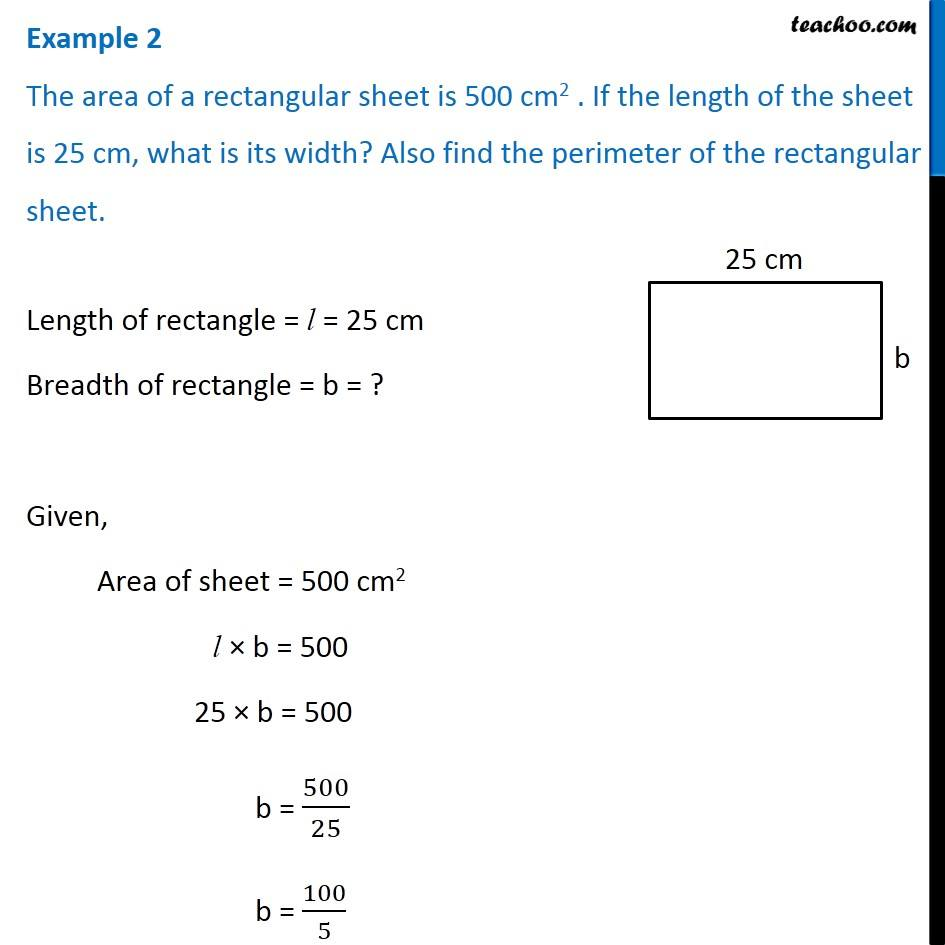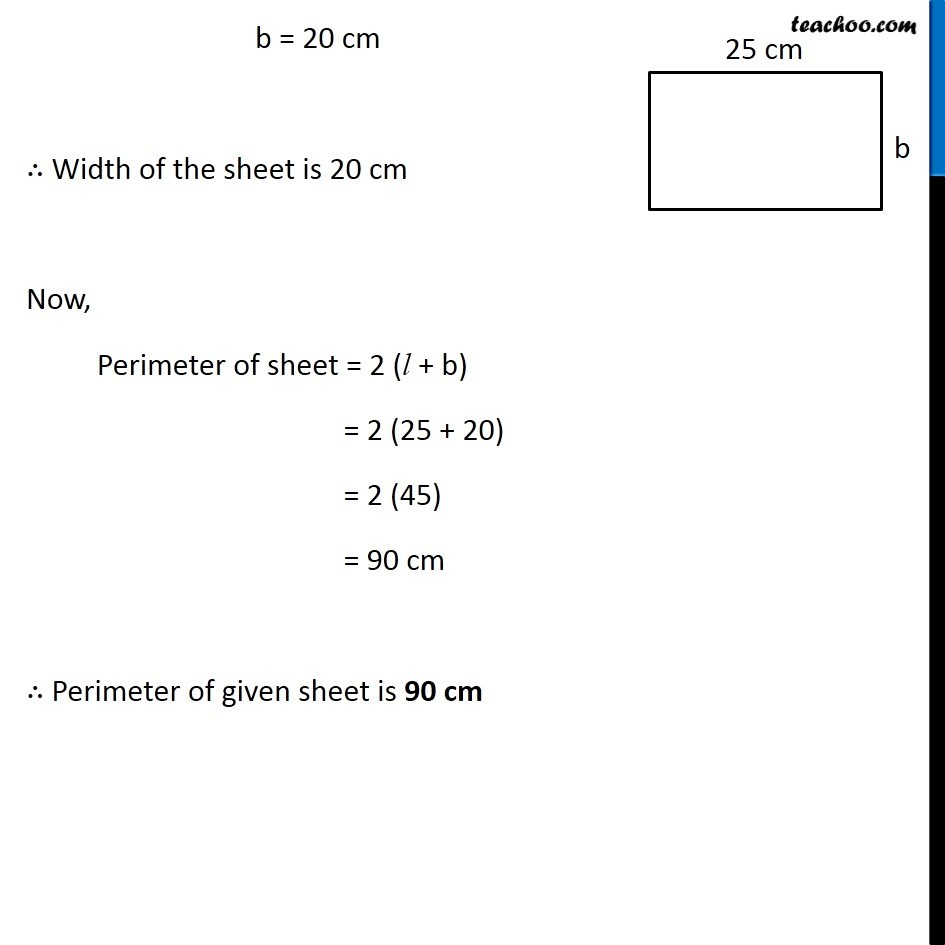Examples

Chapter 9 Class 7 Perimeter and Area
Serial order wiseLearn in your speed, with individual attention - Teachoo Maths 1-on-1 Class

### Transcript

Question 2 The area of a rectangular sheet is 500 cm2 . If the length of the sheet is 25 cm, what is its width? Also find the perimeter of the rectangular sheet. Length of rectangle = l = 25 cm Breadth of rectangle = b = ? Given, Area of sheet = 500 cm2 l × b = 500 25 × b = 500 b = 500/25 b = 100/5 b = 20 cm ∴ Width of the sheet is 20 cm Now, Perimeter of sheet = 2 (l + b) = 2 (25 + 20) = 2 (45) = 90 cm ∴ Perimeter of given sheet is 90 cm